This article demonstrates how to use a linear gradient brush in Silverlight using XAML and C#.

This article demonstrates how to use a linear gradient brush in Silverlight using XAML and C#.

A linear gradient brush paints an area with a linear gradient. The LinearGradientBrush object represents a linear gradient brush. The default value linear gradient value is diagonal. The StartPoint and EndPoint properties of the LinearGradientBrush represent the start and end points of a gradient. The default values of these properties is (0, 0) and (1, 1), which is upper-left corner to lower-right corner of an area.

Figure 1 taken from MSDN shows a rectangle filled with a diagonal gradient and Figure 2 shows a rectangle filled with a gradient brush with multiple gradient stops.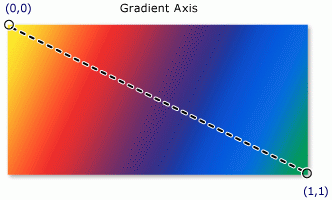Figure 1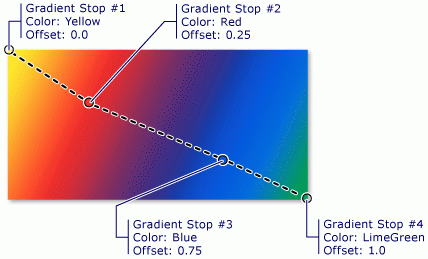Figure 2

Creating a Linear Gradient Brush

The LinearGradientBrush element in XAML creates a linear gradient brush. The following code snippet creates a linear gradient brush with blue and red colors by setting GradientStops. The StartPoint and EndPoint values are (0, 0) and (1, 1).

<LinearGradientBrush StartPoint="0,0" EndPoint="1,1" >

<GradientStop Color="Blue" Offset="0" />

<GradientStop Color="Red" Offset="1.0" />

We can fill a shape with a gradient brush by setting a shape's Fill property to the gradient brush. The code snippet in Listing 1 creates a rectangle shape sets the Fill property to a LinearGradientBrush with blue and red colors.

<Rectangle Width="200" Height="100">

<Rectangle.Fill>

<LinearGradientBrush StartPoint="0,0" EndPoint="1,1" >

<GradientStop Color="Blue" Offset="0" />

<GradientStop Color="Red" Offset="1.0" />

</Rectangle.Fill>

</Rectangle>

Listing 1

The output looks like Figure 3.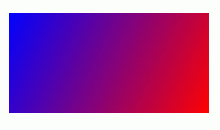Figure 3

Now let's apply multiple stops with multiple colors. The code snippet in Listing 2 creates a linear gradient brush with five stops.

<Rectangle Width="200" Height="100">

<Rectangle.Fill>

<LinearGradientBrush StartPoint="0,0" EndPoint="1,1" >

<GradientStop Color="Blue" Offset="0.1" />

<GradientStop Color="Orange" Offset="0.25" />

<GradientStop Color="Yellow" Offset="0.50" />

<GradientStop Color="Green" Offset="0.75" />

<GradientStop Color="Red" Offset="1.0" />

</Rectangle.Fill>

</Rectangle>

Listing 2

The new output generated by Listing 2 looks like Figure 4.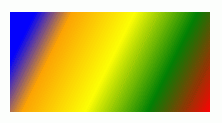Figure 4

The CreateARectangleWithLGBrush method listed in Listing 3 draws same rectangle in Figure 4 dynamically.

public void CreateARectangleWithLGBrush()

{

// Create a Rectangle

Rectangle blueRectangle = new Rectangle();

blueRectangle.Height = 100;

blueRectangle.Width = 200;

// Create a linear gradient brush with five stops

fiveColorLGB.StartPoint = new Point(0, 0);

fiveColorLGB.EndPoint = new Point(1, 1);

blueGS.Color = Colors.Blue;

blueGS.Offset = 0.0;

orangeGS.Color = Colors.Orange;

orangeGS.Offset = 0.25;

yellowGS.Color = Colors.Yellow;

yellowGS.Offset = 0.50;

greenGS.Color = Colors.Green;

greenGS.Offset = 0.75;

redGS.Color = Colors.Red;

redGS.Offset = 1.0;

// Set Fill property of rectangle

blueRectangle.Fill = fiveColorLGB;

// Add Rectangle to the page

}

Listing 3

By simply changing the StartPoint and EndPoint values, we can generate a vertical gradient shapes. By changing a few lines below in code listed in Listing 4 generates Figure 5.

// Create a linear gradient brush with five stops

fiveColorLGB.StartPoint = new Point(0, 0.5);

fiveColorLGB.EndPoint = new Point(1, 0.5);

Listing 4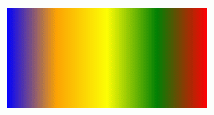Figure 5

Summary

In this article, we saw how to create and use a linear gradient brush in Silverlight using XAML and C#.